Neural Network Diagram shows a two-layer neural network with three X variables and one Y variable. In this example, the first layer has two nodes, and each node is a function of all three nodes in the second layer. The second layer has three nodes, and all nodes are a function of the three X variables. The predicted Y variable is a function of both nodes in the first layer.
Neural Network Diagram
To launch the Neural platform, select Analyze > Modeling > Neural.
The Neural Launch Window
 Y, Response Choose the response variable. When multiple responses are specified, the models for the responses share all parameters in the hidden layers (those parameters not connected to the responses). X, Factor Choose the input variables. Freq Choose a frequency variable. Validation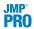Choose a validation column. For more information, see Validation Method. If you click the Validation button with no columns selected in the Select Columns list, you can add a validation column to your data table. For more information about the Make Validation Column utility, see Basic Analysis. By Choose a variable to create separate models for each level of the variable. Missing Value CodingCheck this box to enable informative coding of missing values. This coding allows estimation of a predictive model despite the presence of missing values. It is useful in situations where missing data are informative. If this option is not checked, rows with missing values are ignored. For a continuous variable, missing values are replaced by the mean of the variable. Also, a missing value indicator, named  Is Missing, is created and included in the model. If a variable is transformed using the Transform Covariates fitting option on the Model Launch window, missing values are replaced by the mean of the transformed variable. For a categorical variable, missing values are treated as a separate level of that variable.
The Model Launch Dialog
 Validation Method Hidden Layer Structure or Hidden Nodes BoostingFitting OptionsGo Fits the neural network model and shows the model reports.
After you click Go to fit a model, you can reopen the Model Launch Dialog and change the settings to fit another model.
 • applies a penalty on the model parameters
 • uses an independent data set to assess the predictive power of the model
 • The training set is the part that estimates model parameters.
 • The validation set is the part that estimates the optimal value of the penalty, and assesses or validates the predictive ability of the model.
 • The test set is a final, independent assessment of the model’s predictive ability. The test set is available only when using a validation column. See Validation Methods.
 • If the column has three unique values, then:
 ‒ the smallest value is used for the Training set.
 ‒ the middle value is used for the Validation set.
 ‒ the largest value is used for the Test set.
 • If the column has two unique values, then only Training and Validation sets are used.
 • If the column has more than three unique values, then KFold validation is performed.
 TanH The hyperbolic tangent function is a sigmoid function. TanH transforms values to be between -1 and 1, and is the centered and scaled version of the logistic function. The hyperbolic tangent function is: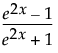where x is a linear combination of the X variables. Linear The identity function. The linear combination of the X variables is not transformed. The Linear activation function is most often used in conjunction with one of the non-linear activation functions. In this case, the Linear activation function is placed in the second layer, and the non-linear activation functions are placed in the first layer. This is useful if you want to first reduce the dimensionality of the X variables, and then have a nonlinear model for the Y variables. For a continuous Y variable, if only Linear activation functions are used, the model for the Y variable reduces to a linear combination of the X variables. For a nominal or ordinal Y variable, the model reduces to a logistic regression. Gaussian The Gaussian function. Use this option for radial basis function behavior, or when the response surface is Gaussian (normal) in shape. The Gaussian function is: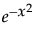where x is a linear combination of the X variables.
The learning rate must be 0 < r 1. Learning rates close to 1 result in faster convergence on a final model, but also have a higher tendency to overfit data. Use learning rates close to 1 when a small Number of Models is specified.
Fitting Options describes the model fitting options that you can specify.
 Transform Covariates Transforms all continuous variables to near normality using either the Johnson Su or Johnson Sb distribution. Transforming the continuous variables helps to mitigate the negative effects of outliers or heavily skewed distributions. Robust Fit Trains the model using least absolute deviations instead of least squares. This option is useful if you want to minimize the impact of response outliers. This option is available only for continuous responses. Penalty Method Number of Tours Specify the number of times to restart the fitting process, with each iteration using different random starting points for the parameter estimates. The iteration with the best validation statistic is chosen as the final model.
The penalty is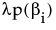, where λ is the penalty parameter, and p( ) is a function of the parameter estimates, called the penalty function. Validation is used to find the optimal value of the penalty parameter.
 Method Penalty Function Description Squared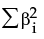Use this method if you think that most of your X variables are contributing to the predictive ability of the model. Absolute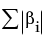Use either of these methods if you have a large number of X variables, and you think that a few of them contribute more than others to the predictive ability of the model. Weight Decay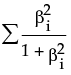NoPenalty none Does not use a penalty. You can use this option if you have a large amount of data and you want the fitting process to go quickly. However, this option can lead to models with lower predictive performance than models that use a penalty.